﻿ Computer Process System Engineering: Courses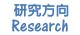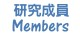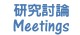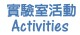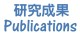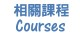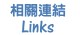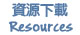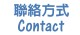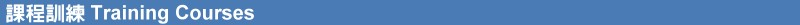• Process Simulation & Optimization Techniques

• (Spring 2019) Class Meeting Times & Locations:
• SAT (010:00~12:30, 工504)
• Office hours: MON (12:00~14:00) and WED (10:00~12:00)
• Optimization Techniques
• (03-01-2019): Introduction
• Problem Formulation
• Design Variables (Continuous, Discrete, Integer or Mixed)
• Design Parameters
• Design Function (Objective, Equality & Inequality, Side Constraints)
• Linear & Nonlinear Programming,  Discrete Programming or Mixed
• Examples:
• Design of a new beverage can
• Design of motherboards of a manufacturing plant
• Solving a differential equation using optimization
• (03-09-2019):: Graphical Optimization
• MATLAB Graphics Functions
• Python Graphcs Functions
• (03-16-2019): Linear Programming
• Problem Definition
• Graphical Solution
• Simplex Method
• (03-23-2019): Linear Programming
• Simplex Method
• Primal and Dual Problem
• Sensitivity Analysis
• (04-01-2019)
• Nonlinear Programming
• Symbolic Computation
• Taylor's Theorem
• (04-8-2019)
• Assignment 1 (Discussion)
• (04-15-2019)
• Nonlinear Programming: Analytical Condition Approach
• Unconstrained
• Equality-Constrained
• Inequality-Constrained
• (04-22-2019)
• Nonlinear Programming: Numerical Approach (One-dimensional problem)
• Necessary and Sufficient Conditsions
• Netwon-Raphson Technique
• Bisection Technique
• Polynomial Approximation
• Golden Section Method
• (04-29-2019)
• Nonlinear Programming (Unconstrained Opt.)
• (Nongradient-Based ) Scan and Zoom
• (Nongradient-Based ) Random Walk
• (Nongradient-Based ) Pattern Seraph
• (Nongradient-Based ) Powell's Method
• (Gradient-Based ) Steepest Descent Method